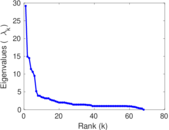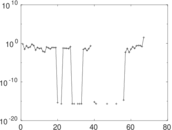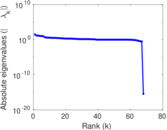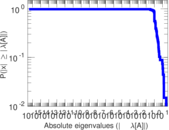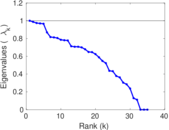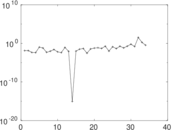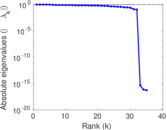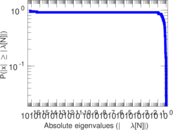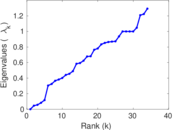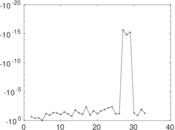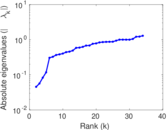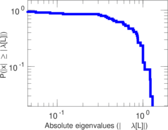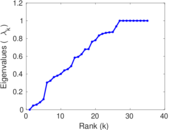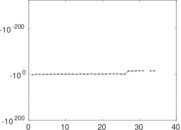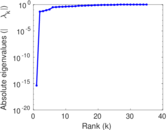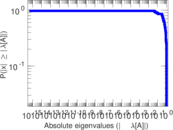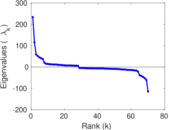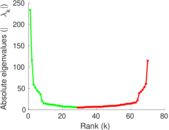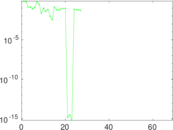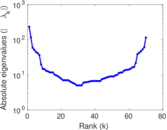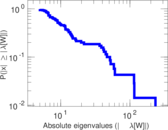# Wikipedia edits (hz)

This is the bipartite edit network of the Herero Wikipedia. It contains users and pages from the Herero Wikipedia, connected by edit events. Each edge represents an edit. The dataset includes the timestamp of each edit.

 Code `hz` Internal name `edit-hzwiki` Name Wikipedia edits (hz) Data source http://dumps.wikimedia.org/ AvailabilityDataset is available for download Consistency checkDataset passed all tests Category Authorship network Dataset timestamp 2017-10-20 Node meaning User, article Edge meaning Edit Network formatBipartite, undirected Edge typeUnweighted, multiple edges Temporal dataEdges are annotated with timestamps

## Statistics

 Size n = 245 Left size n1 = 70 Right size n2 = 175 Volume m = 457 Unique edge count m̿ = 266 Wedge count s = 2,713 Claw count z = 34,311 Cross count x = 365,668 Square count q = 846 4-Tour count T4 = 18,404 Maximum degree dmax = 86 Maximum left degree d1max = 86 Maximum right degree d2max = 73 Average degree d = 3.730 61 Average left degree d1 = 6.528 57 Average right degree d2 = 2.611 43 Fill p = 0.021 714 3 Average edge multiplicity m̃ = 1.718 05 Size of LCC N = 142 Diameter δ = 11 50-Percentile effective diameter δ0.5 = 3.442 66 90-Percentile effective diameter δ0.9 = 5.859 41 Median distance δM = 4 Mean distance δm = 4.053 87 Gini coefficient G = 0.597 261 Balanced inequality ratio P = 0.275 711 Left balanced inequality ratio P1 = 0.210 066 Right balanced inequality ratio P2 = 0.330 416 Relative edge distribution entropy Her = 0.885 632 Power law exponent γ = 3.414 87 Tail power law exponent γt = 2.331 00 Tail power law exponent with p γ3 = 2.331 00 p-value p = 0.857 000 Left tail power law exponent with p γ3,1 = 1.881 00 Left p-value p1 = 0.126 000 Right tail power law exponent with p γ3,2 = 4.561 00 Right p-value p2 = 0.132 000 Degree assortativity ρ = −0.166 868 Degree assortativity p-value pρ = 0.006 374 37 Spectral norm α = 29.169 1 Algebraic connectivity a = 0.045 378 1 Spectral separation |λ1[A] / λ2[A]| = 1.955 71 Controllability C = 108 Relative controllability Cr = 0.446 281

## Plots

### Fruchterman–Reingold graph drawing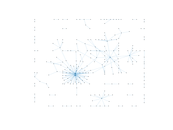### Degree distribution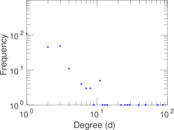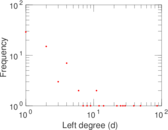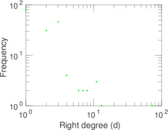### Cumulative degree distribution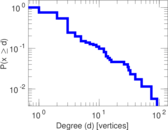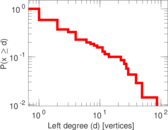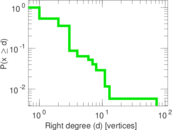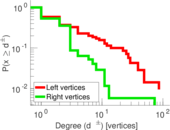### Lorenz curve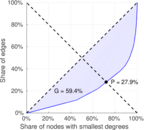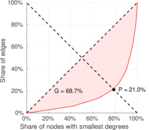### Spectral distribution of the adjacency matrix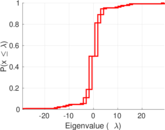### Spectral distribution of the normalized adjacency matrix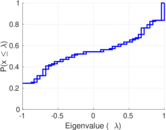### Spectral distribution of the Laplacian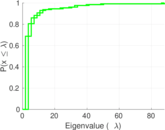### Spectral graph drawing based on the adjacency matrix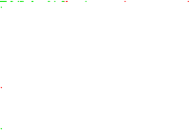### Spectral graph drawing based on the Laplacian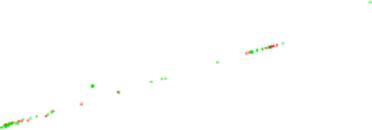### Spectral graph drawing based on the normalized adjacency matrix### Degree assortativity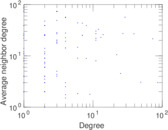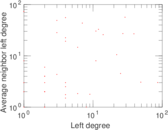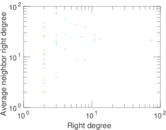### Zipf plot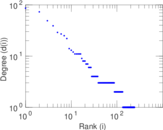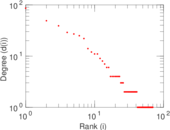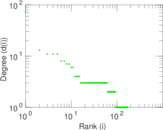### Hop distribution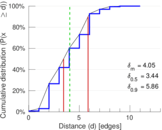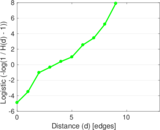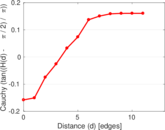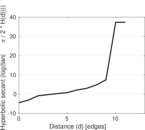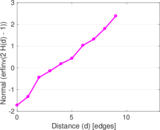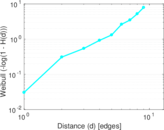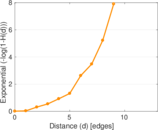### Double Laplacian graph drawing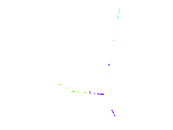### Delaunay graph drawing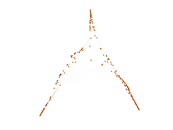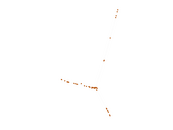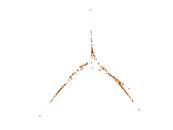### Edge weight/multiplicity distribution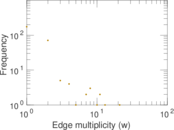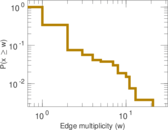### Temporal distribution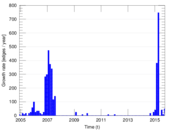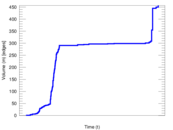### Temporal hop distribution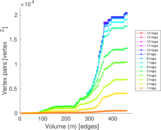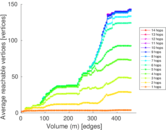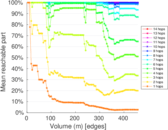### Diameter/density evolution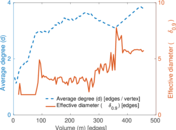### Matrix decompositions plots